# Selina Solutions Concise Mathematics Class 6 Chapter 5: Natural Numbers And Whole Numbers Exercise 5(A)

Exercise 5(A) of Selina Solutions Class 6 contains problems based on the properties of Whole Numbers. Students who aim to improve their skills are advised to go through these solutions in depth. The answers are designed by experts, as per students’ understanding capacity. In order to clear their doubts, students are suggested to practice Selina Solutions regularly. From the links mentioned below, students can access Selina Solutions Concise Mathematics Class 6 Chapter 5 Natural Numbers And Whole Numbers Exercise 5(A) PDF

## Selina Solutions Concise Mathematics Class 6 Chapter 5 Natural Numbers And Whole Numbers Exercise 5(A) Download PDF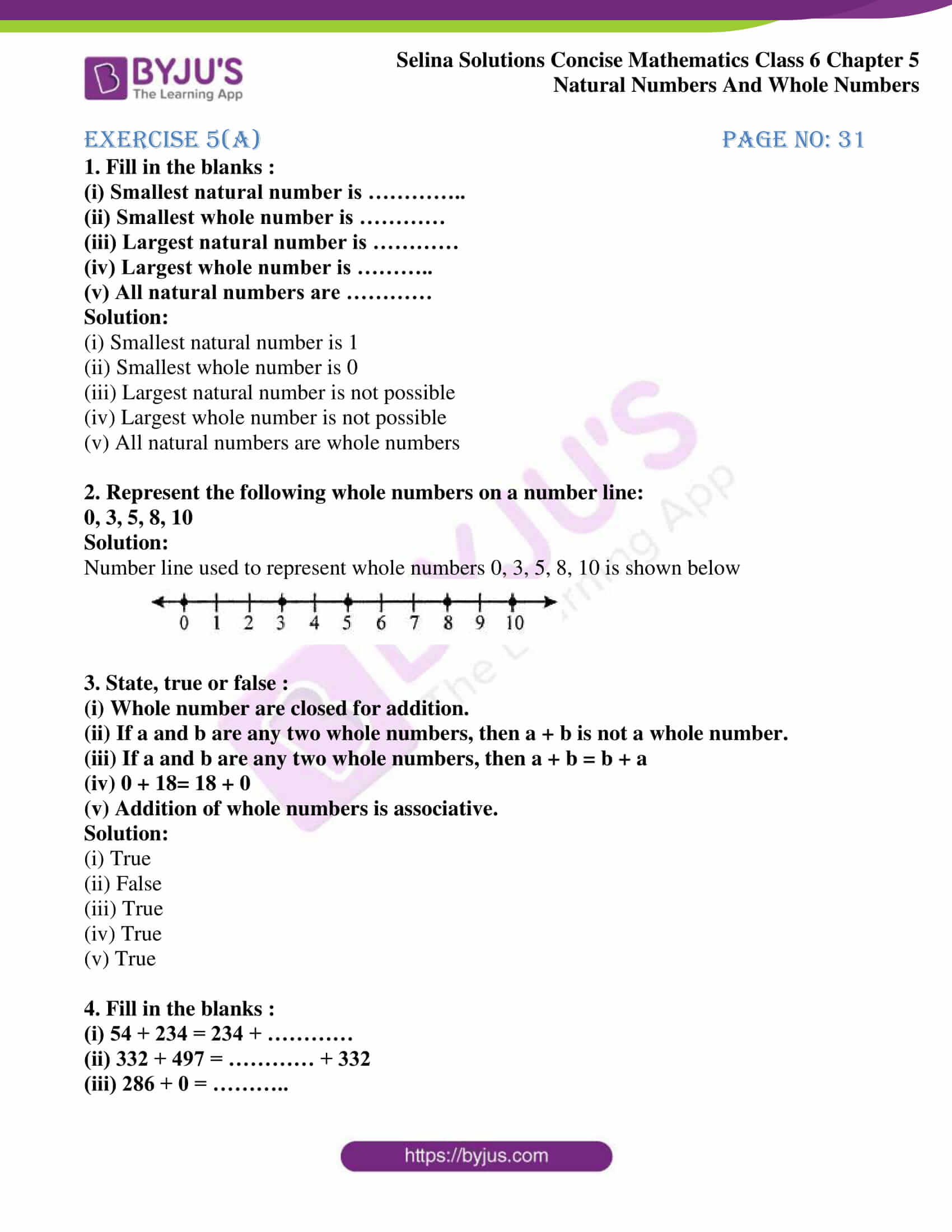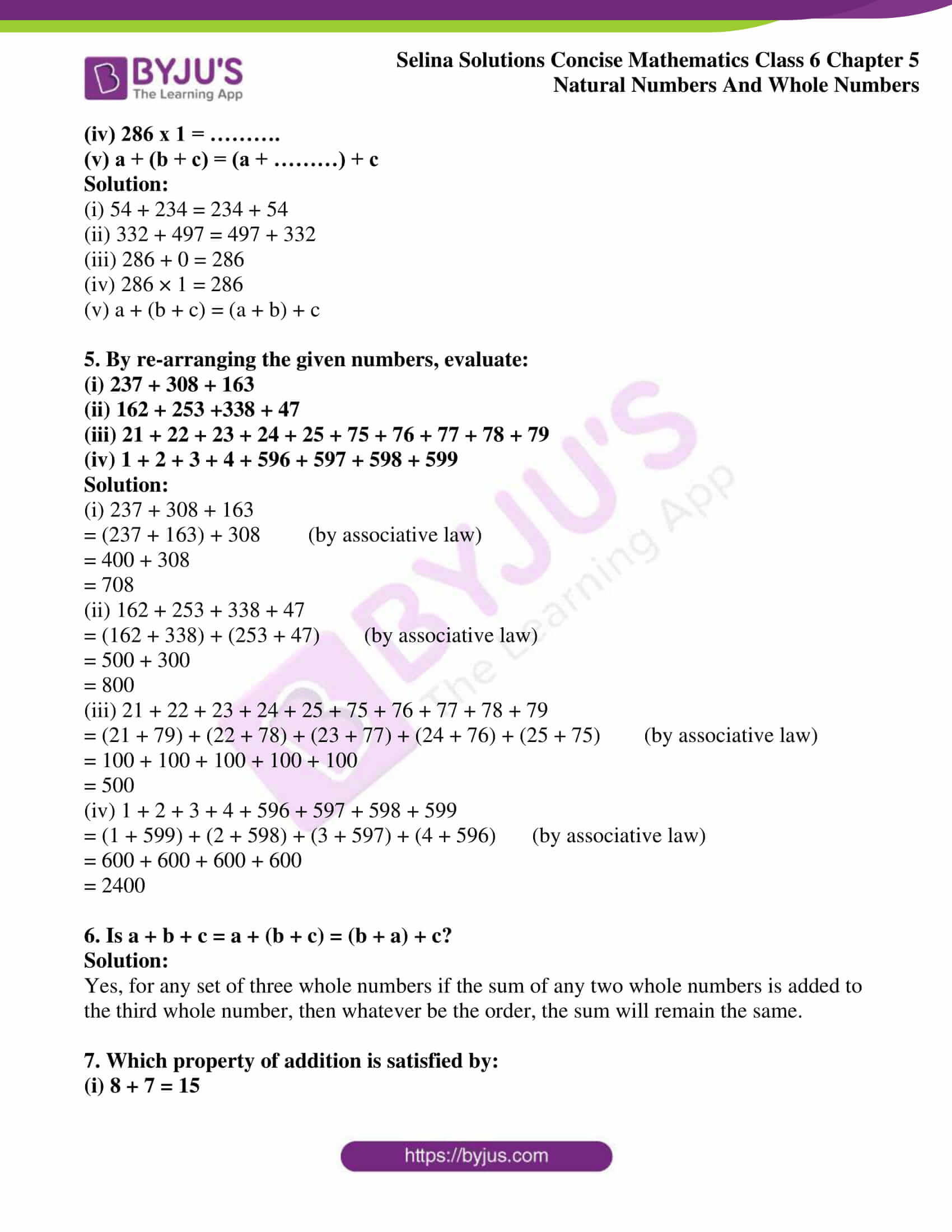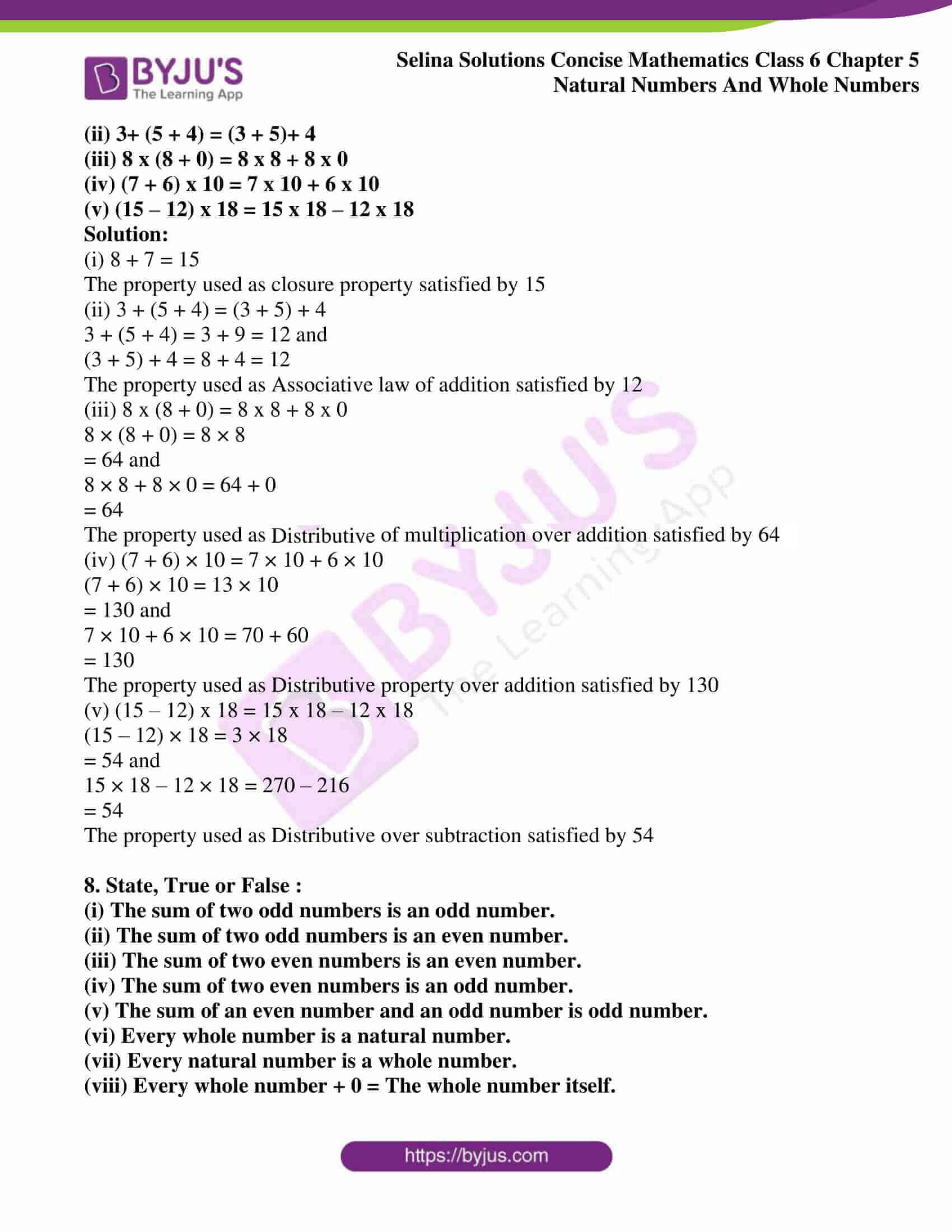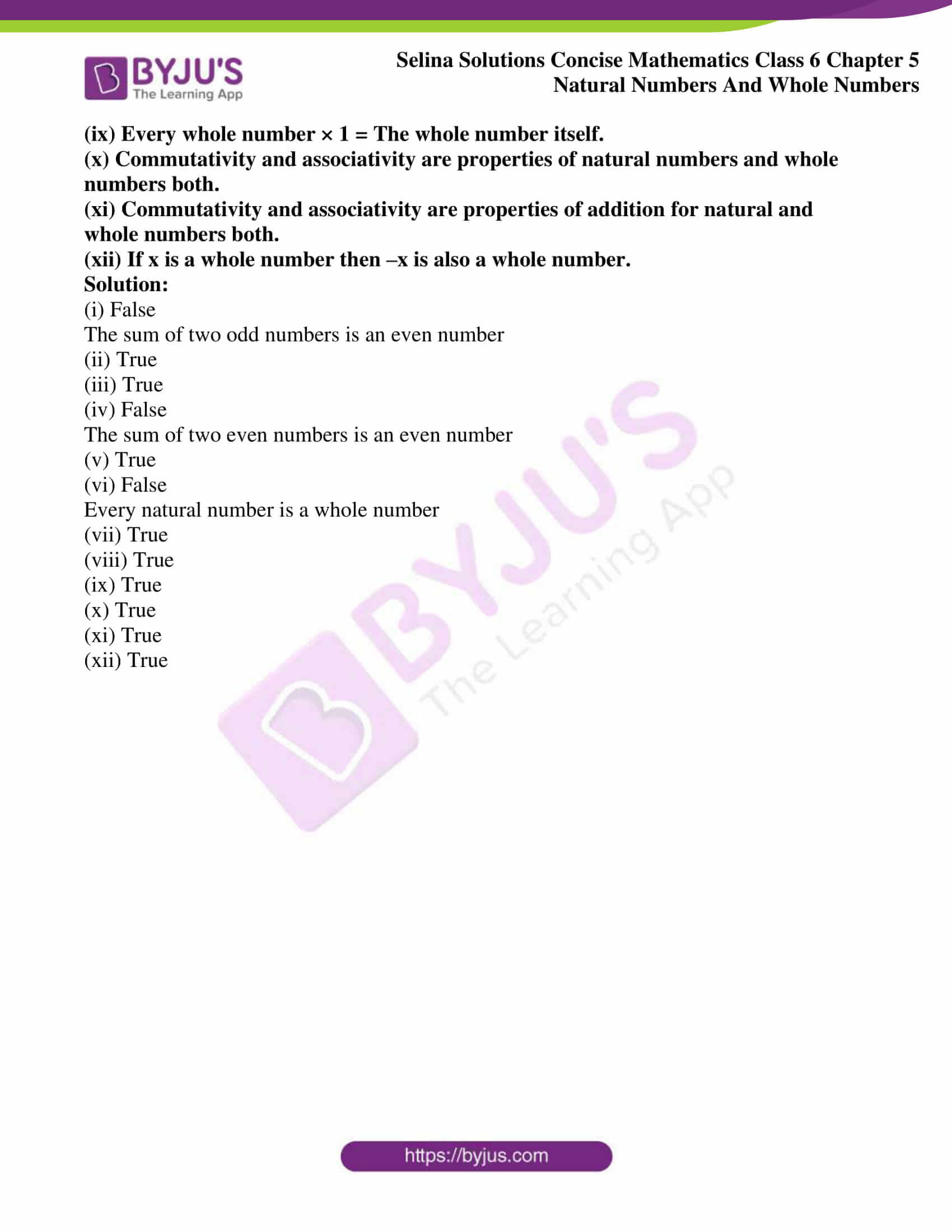### Access other exercises of Selina Solutions Concise Mathematics Class 6 Chapter 5 Natural Number And Whole Numbers

Exercise 5(B) Solutions

Exercise 5(C) Solutions

Exercise 5(D) Solutions

Exercise 5(E) Solutions

Exercise 5(F) Solutions

### Access Selina Solutions Concise Mathematics Class 6 Chapter 5 Natural Numbers And Whole Numbers Exercise 5(A)

#### Exercise 5(A) page no: 31

1. Fill in the blanks :
(i) Smallest natural number is …………..
(ii) Smallest whole number is …………
(iii) Largest natural number is …………
(iv) Largest whole number is ………..
(v) All natural numbers are …………

Solution:

(i) Smallest natural number is 1

(ii) Smallest whole number is 0

(iii) Largest natural number is not possible

(iv) Largest whole number is not possible

(v) All natural numbers are whole numbers

2. Represent the following whole numbers on a number line:
0, 3, 5, 8, 10

Solution:

Number line used to represent whole numbers 0, 3, 5, 8, 10 is shown below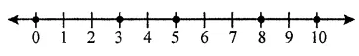3. State, true or false :
(i) Whole number are closed for addition.
(ii) If a and b are any two whole numbers, then a + b is not a whole number.
(iii) If a and b are any two whole numbers, then a + b = b + a
(iv) 0 + 18= 18 + 0
(v) Addition of whole numbers is associative.
Solution:

(i) True

(ii) False

(iii) True

(iv) True

(v) True

4. Fill in the blanks :
(i) 54 + 234 = 234 + …………
(ii) 332 + 497 = ………… + 332
(iii) 286 + 0 = ………..
(iv) 286 x 1 = ……….
(v) a + (b + c) = (a + ………) + c

Solution:

(i) 54 + 234 = 234 + 54

(ii) 332 + 497 = 497 + 332

(iii) 286 + 0 = 286

(iv) 286 × 1 = 286

(v) a + (b + c) = (a + b) + c

5. By re-arranging the given numbers, evaluate:
(i) 237 + 308 + 163
(ii) 162 + 253 +338 + 47
(iii) 21 + 22 + 23 + 24 + 25 + 75 + 76 + 77 + 78 + 79
(iv) 1 + 2 + 3 + 4 + 596 + 597 + 598 + 599

Solution:

(i) 237 + 308 + 163

= (237 + 163) + 308 (by associative law)

= 400 + 308

= 708

(ii) 162 + 253 + 338 + 47

= (162 + 338) + (253 + 47) (by associative law)

= 500 + 300

= 800

(iii) 21 + 22 + 23 + 24 + 25 + 75 + 76 + 77 + 78 + 79

= (21 + 79) + (22 + 78) + (23 + 77) + (24 + 76) + (25 + 75) (by associative law)

= 100 + 100 + 100 + 100 + 100

= 500

(iv) 1 + 2 + 3 + 4 + 596 + 597 + 598 + 599

= (1 + 599) + (2 + 598) + (3 + 597) + (4 + 596) (by associative law)

= 600 + 600 + 600 + 600

= 2400

6. Is a + b + c = a + (b + c) = (b + a) + c?

Solution:

Yes, for any set of three whole numbers if the sum of any two whole numbers is added to the third whole number, then whatever be the order, the sum will remain the same.

7. Which property of addition is satisfied by:
(i) 8 + 7 = 15
(ii) 3+ (5 + 4) = (3 + 5)+ 4
(iii) 8 x (8 + 0) = 8 x 8 + 8 x 0
(iv) (7 + 6) x 10 = 7 x 10 + 6 x 10
(v) (15 – 12) x 18 = 15 x 18 – 12 x 18

Solution:

(i) 8 + 7 = 15

The property used as closure property satisfied by 15

(ii) 3 + (5 + 4) = (3 + 5) + 4

3 + (5 + 4) = 3 + 9 = 12 and

(3 + 5) + 4 = 8 + 4 = 12

The property used as Associative law of addition satisfied by 12

(iii) 8 x (8 + 0) = 8 x 8 + 8 x 0

8 × (8 + 0) = 8 × 8

= 64 and

8 × 8 + 8 × 0 = 64 + 0

= 64

The property used as Distributive of multiplication over addition satisfied by 64

(iv) (7 + 6) × 10 = 7 × 10 + 6 × 10

(7 + 6) × 10 = 13 × 10

= 130 and

7 × 10 + 6 × 10 = 70 + 60

= 130

The property used as Distributive property over addition satisfied by 130

(v) (15 – 12) x 18 = 15 x 18 – 12 x 18

(15 – 12) × 18 = 3 × 18

= 54 and

15 × 18 – 12 × 18 = 270 – 216

= 54

The property used as Distributive over subtraction satisfied by 54

8. State, True or False :
(i) The sum of two odd numbers is an odd number.
(ii) The sum of two odd numbers is an even number.
(iii) The sum of two even numbers is an even number.
(iv) The sum of two even numbers is an odd number.
(v) The sum of an even number and an odd number is odd number.

(vi) Every whole number is a natural number.

(vii) Every natural number is a whole number.

(viii) Every whole number + 0 = The whole number itself.

(ix) Every whole number × 1 = The whole number itself.

(x) Commutativity and associativity are properties of natural numbers and whole numbers both.

(xi) Commutativity and associativity are properties of addition for natural and whole numbers both.

(xii) If x is a whole number then –x is also a whole number.

Solution:

(i) False

The sum of two odd numbers is an even number

(ii) True

(iii) True

(iv) False

The sum of two even numbers is an even number

(v) True

(vi) False

Every natural number is a whole number

(vii) True

(viii) True

(ix) True

(x) True

(xi) True

(xii) True